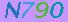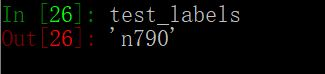# [机器视觉]使用python自动识别验证码详解

更新时间：2019年05月16日 10:13:53   作者：无梦之夜我要评论

CAPTCHA全称Completely Automated Public Turing Test to Tell Computers and Humans Apart，即全自动区分人机的图灵测试。这也是验证码诞生的主要任务。但是随着近年来大数据运算和机器视觉的发展，用机器视觉识别图像已经变得非常容易，过去用于区分人机的验证码也开始变得不再安全。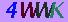```import requests
import time
from io import BytesIO
from PIL import Image
import os
import numpy as np

# 获取验证码的网址
CAPT_URL = "http://xxxxxxxxxxxx.cn/servlet/ImageServlet"

# 验证码的保存路径
CAPT_PATH = "capt/"
if not os.path.exists(CAPT_PATH):
os.mkdir(CAPT_PATH)

# 将验证码转为灰度图时用到的"lookup table"
THRESHOLD = 165
LUT = *THRESHOLD + *(256 - THRESHOLD)
```

capt_fetch()方法非常简单，我们直接从网站获取验证码，将其转换为Image对象，等待被训练和测试等环节调用。

```def capt_fetch():
"""
从网站获取验证码，将验证码转为Image对象

:require requests: import requests
:require time: import time
:require BytesIO: from io import BytesIO
:require Image: from PIL import Image

:param:
:return capt: 一个Image对象
"""
# 从网站获取验证码
capt_raw = requests.get(CAPT_URL)

# 将二进制的验证码图片写入IO流
f = BytesIO(capt_raw.content)

# 将验证码转换为Image对象
capt = Image.open(f)

return capt
```

1. 一个强大的机器学习模型，是离不开强大的训练集作支持的。这里我们也必须先有一个预先打好标签（预分类）的验证码图片集，才能开始训练模型。
2. capt_download()方法就是我们用来建立训练图像集的方法。它会调用capt_fetch()方法，将获得的Image对象展示给用户，等待用户输入验证码中的字符，然后将图片命名为用户输入的字符存储起来。
3. 当然，为了避免文件名重复（比如获取到了两张字符完全相同的验证码），capt_download()方法将系统时间也加入到了文件名中。
```def capt_download():
"""
将Image类型的验证码对象保存到本地

:require Image: from PIL import Image
:require os: import os

:require capt_fetch(): 从nbsc网站获取验证码
:require CAPT_PATH: 验证码保存路径

:param:
:return:
"""
capt = capt_fetch()
capt.show()

text = raw_input("请输入验证码中的字符：")
suffix = str(int(time.time() * 1e3))

capt.save(CAPT_PATH + text + "_" + suffix + ".jpg")
```

图像预处理

1. capt_process()方法会先将验证码转为灰度图，然后再根据全局变量中定义的LUT将灰度图转化为黑白图片。并按照验证码中四个字符所在的位置进行切割。
2. 从彩色图片到灰度图，再到黑白图，看似验证码中的信息损失了很多，实际上这样做的目的是为了使字符的特征更加明显。
3. 其实我们最终得到的黑白图像会有一些噪点存在，这主要是由于前景色与背景色不存在严格的区分度，我们可以使用滤波器过滤掉这些噪点，但少量的噪点会被训练模型当作误差处理，并不影响我们分类。至于过滤噪点的方法，我会专门写一篇帖子。
```def capt_process(capt):
"""
图像预处理：将验证码图片转为二值型图片，按字符切割

:require Image: from PIL import Image
:require LUT: A lookup table, 包含256个值

:param capt: 验证码Image对象
:return capt_per_char_list: 一个数组包含四个元素，每个元素是一张包含单个字符的二值型图片
"""
capt_gray = capt.convert("L")
capt_bw = capt_gray.point(LUT, "1")

capt_per_char_list = []
for i in range(4):
x = 5 + i * 15
y = 2
capt_per_char = capt_bw.crop((x, y, x + 15, y + 18))
capt_per_char_list.append(capt_per_char)

return capt_per_char_list
```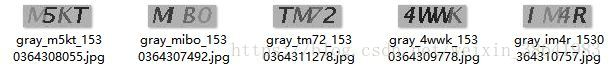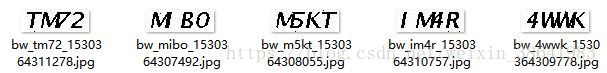1. 到了这一步，我们得到的图像都是由单个字符组成的黑白图片（0为黑色像素点，1为白色像素点）。此时，如果我们把图片转为数组，就会得到一个由0，1组成的矩阵，其长宽恰与图片的大小相同， 每一个数字代表一个像素点。
2. 接下来我们需要考虑如何提取能够区分不同字符的特征值。我们可以直接用图像中的每一个像素点作为一个特征值，也可以汇总图像中共有多少黑色像素点（当然，这样每张图片只能提取一个特征值），还可以按区域汇总图像中的像素点，比如先将图片四等分，汇总四张“子图片”中的像素点，如果觉得特征不够多，还可以继续下分，直至精确到每一个像素点。
3. 为了使代码更加简洁，我们这里直接汇总、分行列汇总了图像像素点的个数，共提取了1张图片 + 15列 + 18行 ==> 34个特征值。至于按区域汇总的方法，还是等我们有空了单独写一篇帖子。
```def capt_inference(capt_per_char):
"""
提取图像特征

:require numpy: import numpy as np

:param capt_per_char: 由单个字符组成的二值型图片
:return char_features:一个数组，包含 capt_per_char中字符的特征
"""
char_array = np.array(capt_per_char)

total_pixels = np.sum(char_array)
cols_pixels = np.sum(char_array, 0)
rows_pixels = np.sum(char_array, 1)

char_features = np.append(cols_pixels, rows_pixels)
char_features = np.append(total_pixels, char_features)

return char_features.tolist()
```

```def train():
"""
将预分类的验证码图片集转化为字符特征训练集

:require Image: from PIL import Image
:require os: import os

:require capt_process(): 图像预处理
:require capt_inference(): 提取图像特征

:param:
:return train_table: 验证码字符特征训练集
:return train_labels: 验证码字符预分类结果
"""
files = os.listdir(CAPT_PATH)

train_table = []
train_labels = []

for f in files:
train_labels += list(f.split("_"))

capt = Image.open(CAPT_PATH + f)
capt_per_char_list = capt_process(capt)
for capt_per_char in capt_per_char_list:
char_features = capt_inference(capt_per_char)
train_table.append(char_features)

return train_table, train_labels
```

1. 只要我们提取的特征值具有足够的区分度（能够区分不同字符），理论上我们可以使用任何机器学习的模型建立特征值与标签的相关。
2. 我尝试过使用knn，svm，Decision Tree，ANN等各种主流机器学习模型对提取的训练数据进行分类，尽管不同模型表现各异，但识别准确率都可以很轻松的达到90%以上。
3. 这里我们不打算使用复杂的第三方模型完成学习过程，所以我们在这里自己写了一个分类模型。模型实现的nnc算法是knn的一种，通过比对未知分类的一组特征值测试集与那一组已知分类的特征值训练集最接近，判定测试集的分类情况应该和与其最接近的训练集的分类情况训练集标签相同。
4. 当然，我们也可以稍加改造直接实现knn算法，找到3组、5组或k组与未知分类的特征向量最接近的训练集中的特征向量，并通过票选与其对应的标签，预测未知分类的特征向量在大概率上应该属于哪一类。
```def nnc(train_table, test_vec, train_labels):
"""
Nearest Neighbour Classification（近邻分类法），
根据已知特征矩阵的分类情况，预测未分类的特征向量所属类别

:require numpy: import numpy as np

:param train_table: 预分类的特征矩阵
:param test_vec: 特征向量， 长度必须与矩阵的列数相等
:param labels: 特征矩阵的类别向量
:return : 预测特征向量所属的类别
"""

dist_mat = np.square(np.subtract(train_table, test_vec))
dist_vec = np.sum(dist_mat, axis = 1)
pos = np.argmin(dist_vec)

return train_labels[pos]
```

测试模型分类效果

```def test():
"""
测试模型分类效果

:require Image: from PIL import Image

:require capt_fetch(): 从nbsc网站获取验证码
:require capt_process(): 图像预处理
:require capt_inference(): 提取图像特征
:train_table, train_labels: train_table, train_labels = train()

:param:
:return capt: 验证码图片
:return test_labels: 验证码识别结果
"""

test_labels = []

capt = capt_fetch()
capt_per_char_list = capt_process(capt)
for capt_per_char in capt_per_char_list:
char_features = capt_inference(capt_per_char)
label = nnc(train_table, char_features, train_labels)
test_labels.append(label)

test_labels = "".join(test_labels)

return capt, test_labels
```

1. 方法具备，接下来就是我们实践的环节了。
2. 首先，我们需要建立一个机器学习库，即一个预分类的验证码图片集。这里我们仅仅获取了120张验证码作为训练集，相比tensorflow动辄成千上万次的迭代，我们建立模型所需的样本量非常之少。当然，这也要感谢我们使用的nnc算法并不需要十分庞大的训练集支持，才使得我们能够节省很多预分类时人工识别验证码的精力。
3. 接下来，我们会调用train()方法生成训练集和训练集标签，这两个数组会被test()方法用到，但我们把这两个数组存储在全局变量里，所以不需要特意传递给test()方法。
```# 下载120张图片到本地
for i in range(120):
capt_download()

# 模型的训练与测试
train_table, train_labels = train()
test_capt, test_labels = test()```